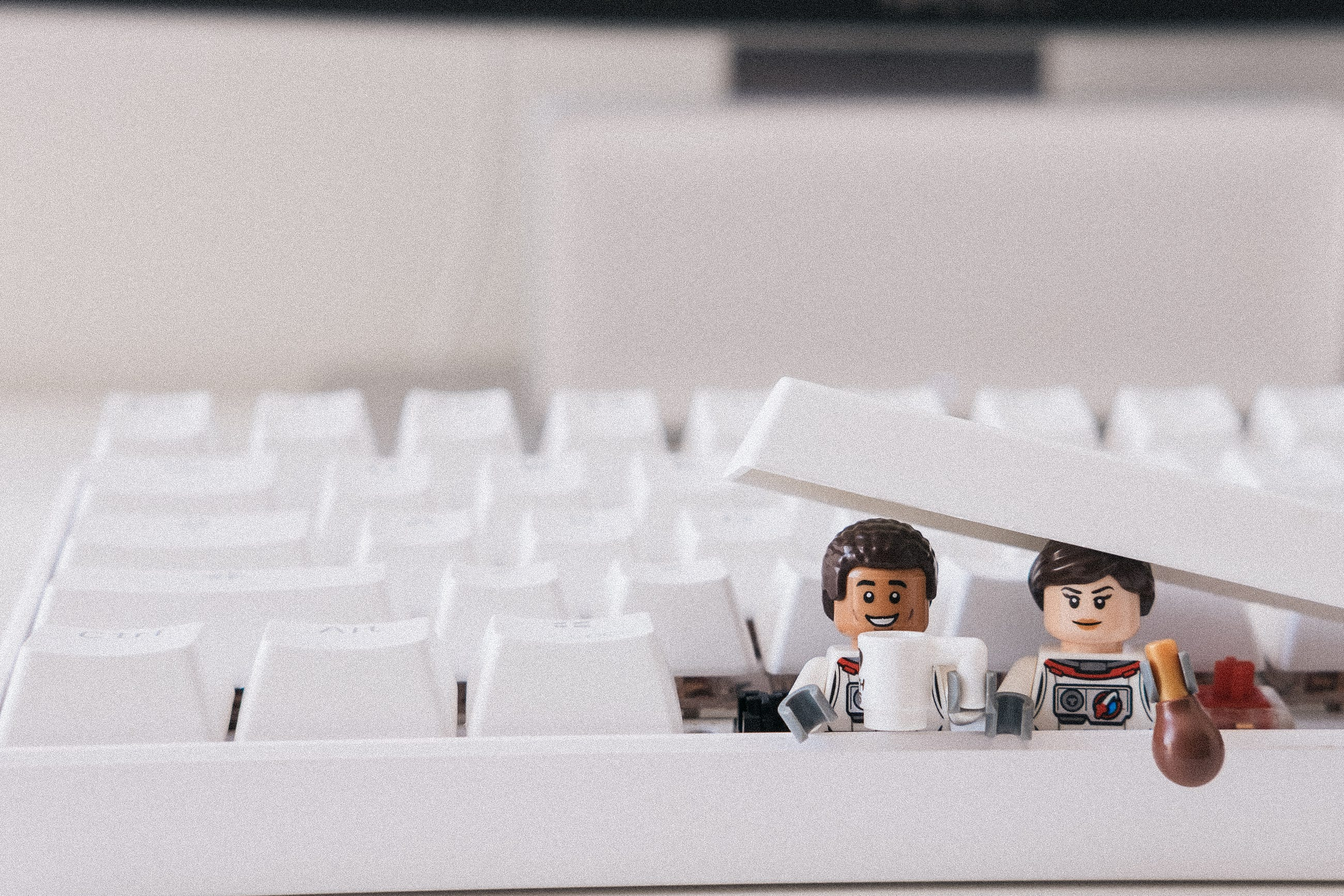# Lego Minifigure Gender Classification Using Deep Learning

Original article was published by Oscar Kwok on Deep Learning on MediumI then generated a download link so that I could use `!wget` and `!unzip` to load the images into Google Colab.

As the images I took had a large size, I needed to transform them so that the input data could work with what the pre-trained model expected (in my case, VGG16). I also used PyTorch’s ImageFolder class so that I could load the data from the train and test folders I created in the zip file.

`data_dir = 'Lego (compressed pics)'# VGG-16 Takes 224x224 images as input, so we resize all of themdata_transform = transforms.Compose([transforms.Resize((224, 224)),                                     transforms.ToTensor()])train_data = datasets.ImageFolder(data_dir + '/Train',              transform=data_transform)test_data = datasets.ImageFolder(data_dir + '/Test',             transform=data_transform)`

Here you can see how I allocated the photos into a train and test set.

Create DataLoaders for the train and test datasets:

`# how many samples per batch to loadbatch_size = 20# number of subprocesses to use for data loadingnum_workers = 0train_loader = torch.utils.data.DataLoader(train_data, batch_size=batch_size, num_workers=num_workers, shuffle=True)test_loader = torch.utils.data.DataLoader(test_data, batch_size=batch_size, num_workers=num_workers, shuffle=True)# specify the image classesclasses = ['Boy', 'Girl']`

Now let’s visualize a batch of training data.

`# obtain one batch of training imagesdataiter = iter(train_loader)images, labels = dataiter.next()images = images.numpy()  # convert images to numpy for display# plot the images in the batch, along with the corresponding labelsfig = plt.figure(figsize=(25,4))for idx in np.arange(20):  ax = fig.add_subplot(2, 20/2, idx+1, xticks=[], yticks=[])     plt.imshow(np.transpose(images[idx], (1, 2, 0)))     ax.set_title(classes[labels[idx]])`